# 堆 堆排序 优先队列

## 堆

• 最大堆（大顶堆）
• 最小堆（小顶堆）

### 最大堆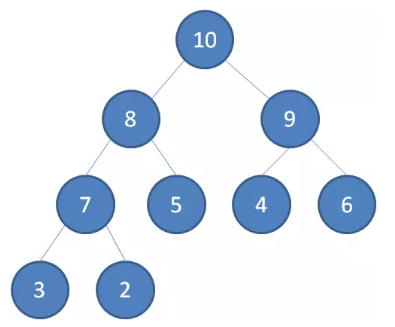### 最小堆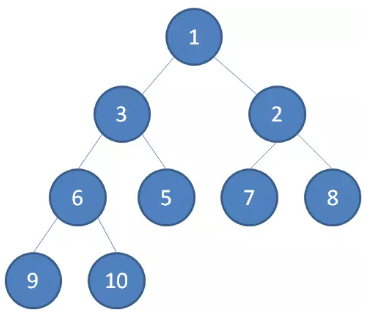## 堆的逻辑结构与物理存储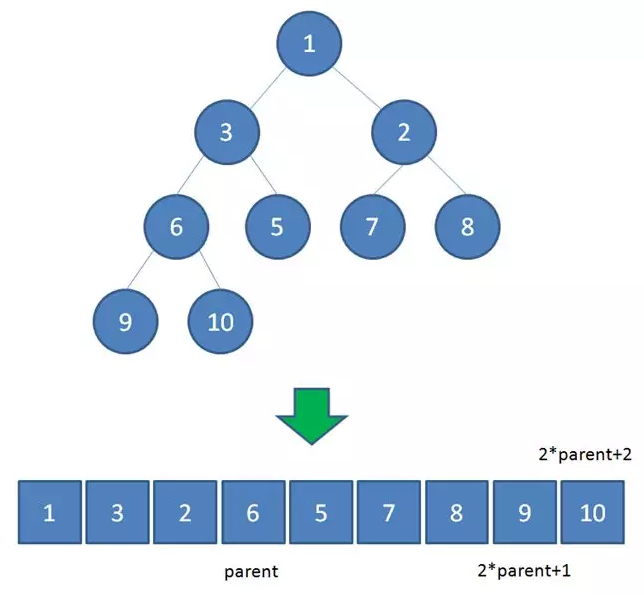• 左儿子的下标 = 2 * parent + 1
• 右儿子的下标 = 2 * parent + 2

• 插入节点 push

• 删除节点 pop

## 堆的自我调整

### 插入节点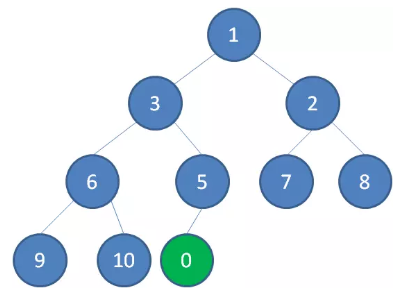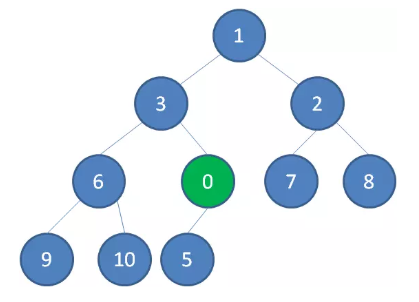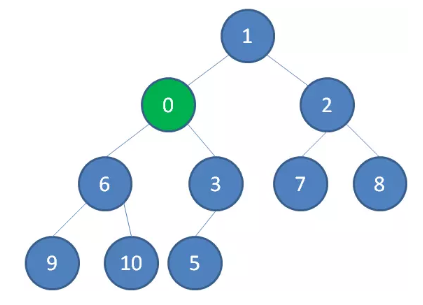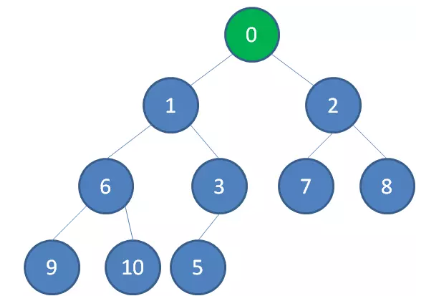### 删除节点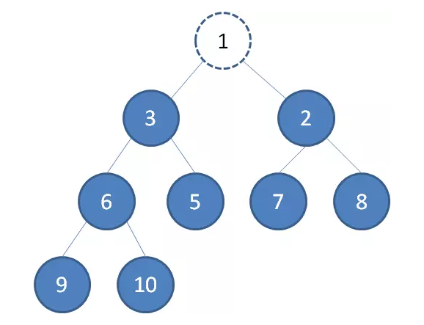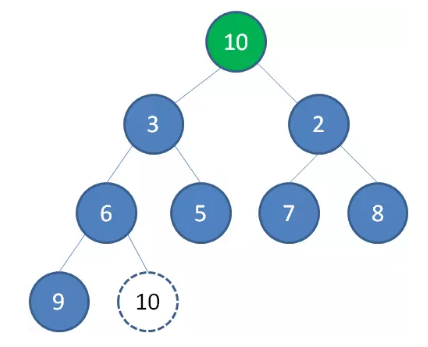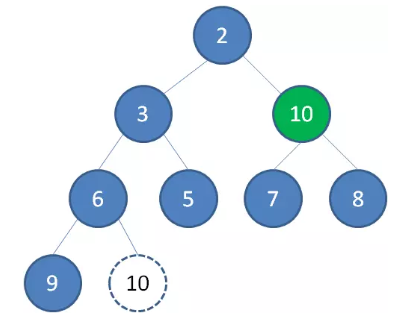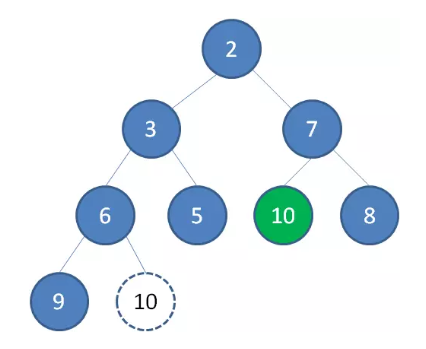## 堆排序

### 构建二叉堆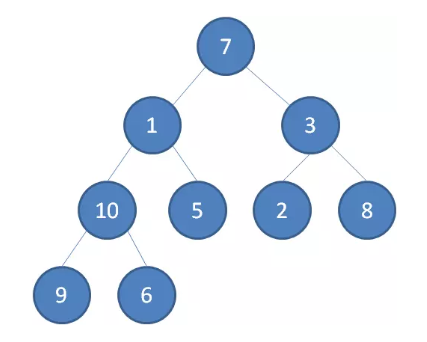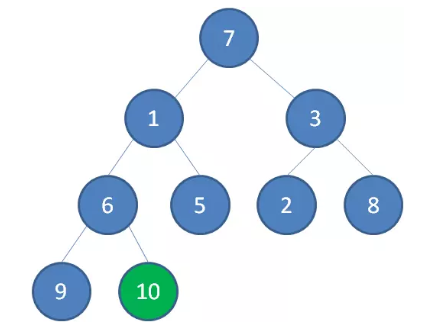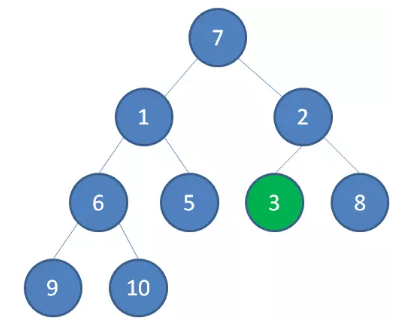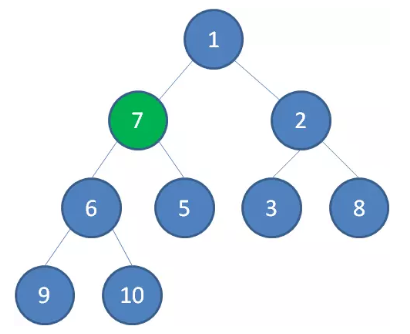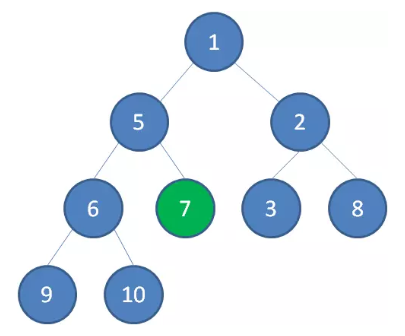### 堆排序过程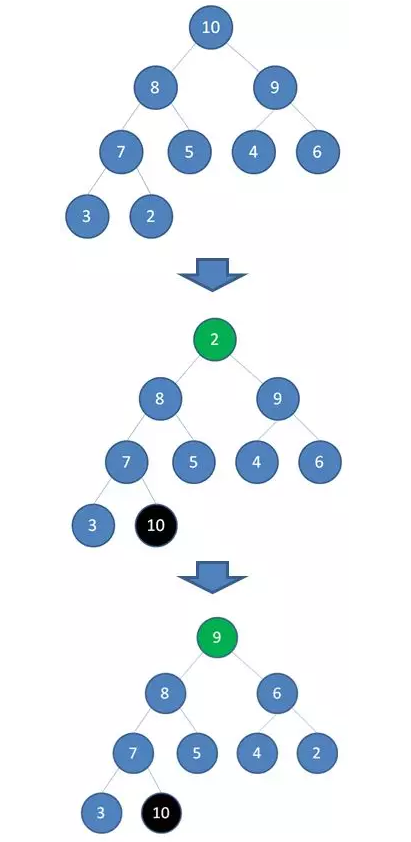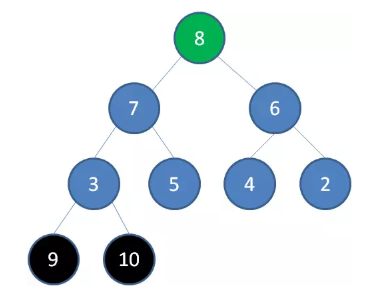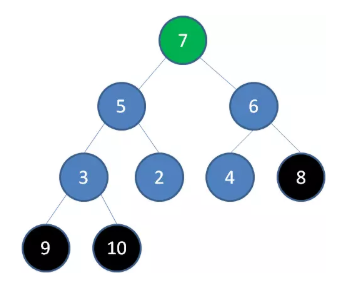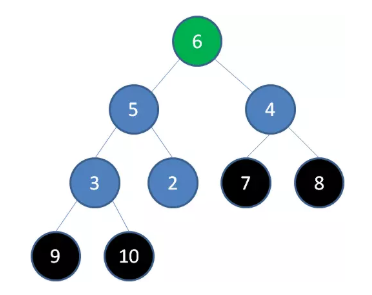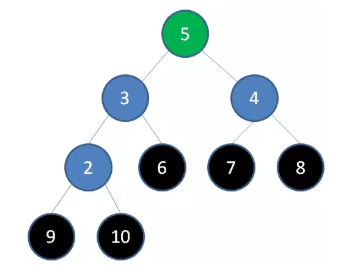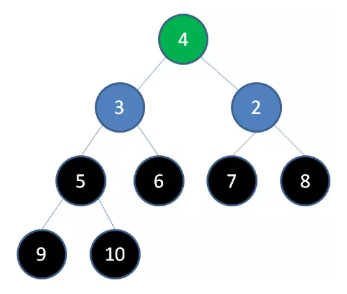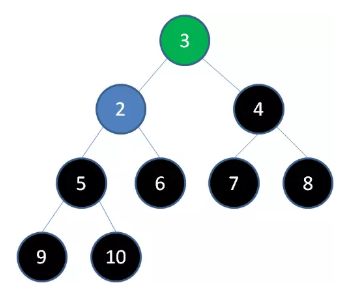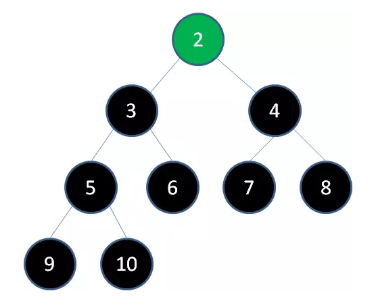### 算法步骤

• 根据无序数组构建二叉堆
• 循环删除堆顶元素，移到数组尾部，调节堆产生新的堆顶

### 堆排序的复杂度

• 空间复杂度

因为没有开辟额外的集合空间，所以空间复杂度为 $$O(1)$$

• 时间复杂度

堆排序所需要的时间主要有两部分组成，一是构建二叉堆的时间，二是循环删除并进行堆维护的时间。

• 第一步，把无序数组构建成二叉堆，需要进行 $$n/2$$ 次循环。每次循环调用一次 sinking 方法，所以第一步的计算规模是 $$(n/2) * logn$$，时间复杂度 $$O(nlogn)$$

这里只是初略地把每个非叶子节点下沉的时间复杂度都当成 $$O(logn)$$ ，事实上经过严格的证明构建堆的时间复杂是 $$O(n)$$ ，但这里我们初略算出的复杂度为 $$O(nlogn)$$ ，当然我们算出的复杂度也是正确的，只不过是不够精确而已。

$$O(n)$$ 的证明也比较简单，可以参考：

https://blog.csdn.net/LinuxTiger/article/details/7172258

• 第二步，需要进行 $$n-1$$ 次循环。每次循环调用一次 sinking 方法，所以第二步的计算规模是 $$(n-1)*logn$$ ，时间复杂度 $$O(nlogn)$$

总的时间复杂度为： $O(n) + O(nlogn) = O(nlogn)$

### 堆排序和快排对比

• 相同点
• 堆排序和快速排序的平均时间复杂度都是 $$O(nlogn)$$
• 两者都是不稳定的排序
• 快速排序因为递归所以需要额外的空间开销 $$O(logn) \sim O(n)$$ ；堆排序是就地排序，空间复杂度 $$O(1)$$
• 不同点
• 堆排序在最好、最坏、平均情况下时间复杂度都是 $$O(nlogn)$$ ；快排在最坏情况下是 $$O(n^2)$$ ，最好情况下是 $$O(nlogn)$$

## 优先队列

### 优先队列种类

• 最大优先队列，无论入队顺序，当前最大的元素优先出队。
• 最小优先队列，无论入队顺序，当前最小的元素优先出队。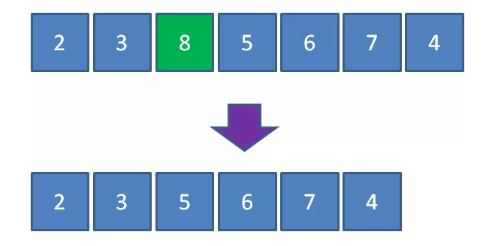### 利用堆的特性实现优先队列

• 最大堆的堆顶是整个堆中的最大元素
• 最小堆的堆顶是整个堆中的最小元素

#### 入队操作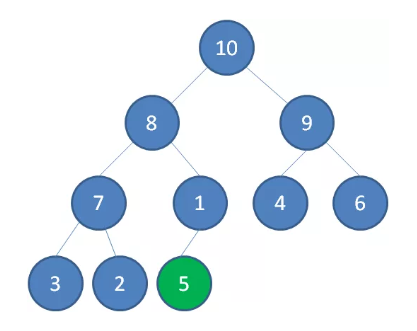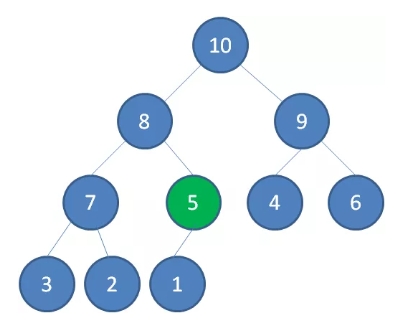#### 出队操作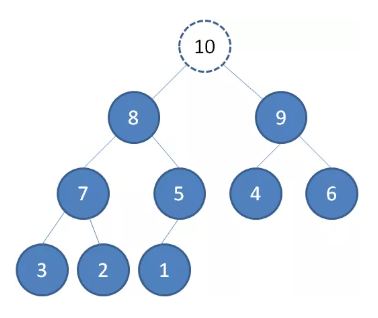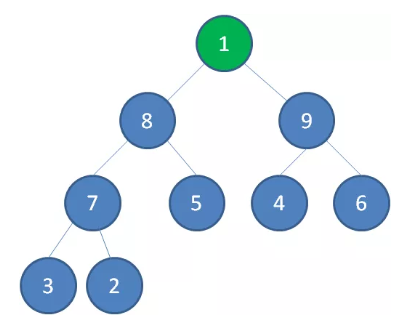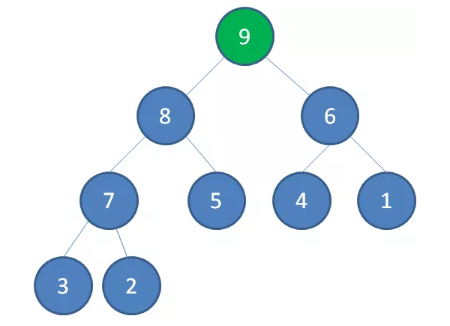### 参考实现

https://en.wikipedia.org/wiki/Heap_(data_structure)

https://mp.weixin.qq.com/s/23CwW5eSv-lI6vaZHzSwhQ

https://mp.weixin.qq.com/s/PWuuChodYGMr8iJzW2VW9Q

https://mp.weixin.qq.com/s/CdK1l2kB3aCUtBORjS4DVQ